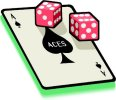edHelper.com Math WorksheetsProbability

Write whether each event is certain, likely, unlikely, or impossible
Probability problems (gives half of the answer)
Probability problems
Probability with spinners (gives half of the answer)
Probability with spinners
Probability Mixed Review

Probability
Counting principle
Theoretical probability (write answer as a fraction)
Theoretical probability (write answer as a decimal)
Theoretical probability (write answer as a percent)
Theoretical probability
Compound events (independent events)
Compound events (dependent events)
Compound events
Combinations
Permutations
Combinations and permutations
Word problems - experimental probability
Word problems - theoretical probability
Word problems - compound events
Word problems - combinations and permutations
Word problems review
Probability Review Printable

Teacher Recommendations:  Worksheet and Lesson Plan Activity Ideas

Math

Word Search

Handwriting

Poetry

Money

Patterns

Geometry

Weather

Plants

Science

Main Idea

Solar System

Writing

More Activities, Lesson Plans, and Worksheets

Have a suggestion or would like to leave feedback?